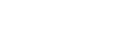## Contribution weights by user

3

(click on this box to dismiss)Q&A for Ubuntu users and developers

``````-- Ranks users by total contribution of characters to the site, including questions, answers, comments,
-- and miscellaneous content such as tag wikis, and provides the total, broken down by category.

select
RANK() OVER
(PARTITION BY 1 ORDER BY sum(case when posttypeid < 100 then weight else 0 end) DESC) AS Rank
, sum(case when posttypeid < 100 then weight else 0 end) as Total_Weight
, round(sum(case when posttypeid < 100 then weight else 0 end) * 100.0 / (p.total + c.total), 2)
as Percent_of_Site_Total
, sum(case when posttypeid = 1 or posttypeid = 2 then weight else 0 end)
as QA_Weight
, round(sum(case when posttypeid = 1 or posttypeid = 2 then weight else 0 end) * 100.0 / p.total, 2)
as Percent_of_QA_Site_Total
, sum(case when posttypeid = 0 then weight else 0 end)
as Comment_Weight
, sum(case when posttypeid > 2 and posttypeid < 100 then weight else 0 end)
as Misc_Weight
, sum(case when posttypeid > 99 then weight else 0 end) as Upvoted_Total_Weight
, sum(case when posttypeid = 101 or posttypeid = 102 then weight else 0 end)
as Upvoted_QA_Weight
, sum(case when posttypeid = 100 then weight else 0 end)
as Upvoted_Comment_Weight
from
(
-- all posts
select
owneruserid
, posttypeid
, sum(len(body)) as weight
from posts
group by owneruserid, posttypeid

union

-- upvoted posts (Type ID += 100)
select
owneruserid
, posttypeid + 100 as posttypeid
, sum(len(body)) as weight
from posts
where score > 0
group by owneruserid, posttypeid

union

-- all comments (Type ID = 0)
select
userid as owneruserid
, 0 as posttypeid
, sum(len(text)) as weight
group by userid

union

-- upvoted comments (Type ID = 100)
select
userid as owneruserid
, 100 as posttypeid
, sum(len(text)) as weight
where score > 0
group by userid
) w

inner join
(select sum(len(body)) as total from posts) p
on 1=1

inner join
(select sum(len(text)) as total from comments) c
on 1=1

group by p.total, c.total, owneruserid

order by sum(case when posttypeid < 100 then weight else 0 end) desc``````

### Enter Parameters

Options:
-Hold tight while we fetch your results
:records returned in :time ms:cached